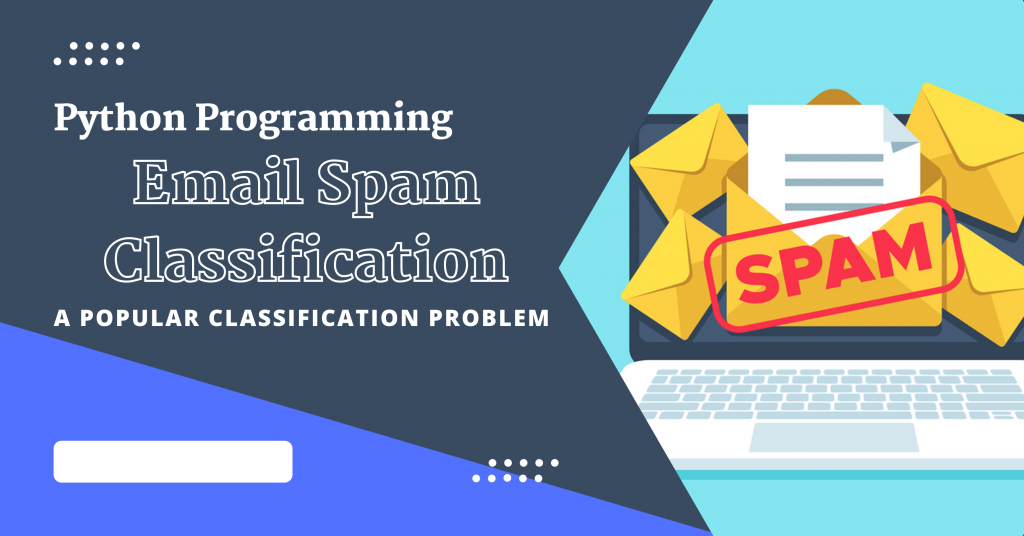# Email Spam Classification in PythonHello fellow learner! In this tutorial, we will talk about how to achieve the classification of spam emails with the help of the dataset which will be loaded using scikit-learn in Python programming language.

## Introduction to Email Spam

We all know that billions of spam are sent every day to user email accounts and more than 90% of these spam emails are malicious and cause major harm to the user.

Don’t the spams get annoying to you as well? They get pretty annoying to me for sure! Sometimes even some important mails get transferred to spam and as a result, some important information is left unread with the fear of getting harmed by the spam emails.

And did you know that one out of every 1,000 e-mails contains malware charges? And hence it is important for us to learn how can we ourselves classify our emails as safe and unsafe.

## Implementing Email Spam Classifier in Python

Let’s get right into the steps to implement an email spam classification algorithm using Python. This will help you understand the backend working of a very basic spam classifier. The algorithms used in the real world are way more advanced compared to the algorithm I’ve described below. But you sure can use this as a starting point for your journey.

First, we import all the necessary required modules into our program. The code for the same is as follows:

```import numpy as np
import pandas as pd
import matplotlib.pyplot as plt

from sklearn.feature_extraction.text import CountVectorizer
from sklearn.naive_bayes import MultinomialNB, GaussianNB
from sklearn import svm
from sklearn.model_selection import GridSearchCV
```

We would require some basic machine learning modules such as numpy, pandas, and matplotlib. Along with these, we would require some `sklearn` models and features.

The next step involves loading the dataset with the help of the pandas module imported earlier. The dataset we would be using is the `spam.csv` data file which can be found here.

```data = pd.read_csv('./spam.csv')
```

The dataset we loaded has 5572 email samples along with 2 unique labels namely, `spam` and `ham`.

### 2. Training and Testing Data

After loading we have to separate the data into training and testing data

The separation of data into training and testing data includes two steps:

1. Separating the x and y data as the email text and labels respectively
2. Splitting the x and y data into four different datasets namely x_train,y_train,x_test, and y_test based on the 80:20 rule.

The separation of data into x and y data is done in the following code:

```x_data=data['EmailText']
y_data=data['Label']

split =(int)(0.8*data.shape)
x_train=x_data[:split]
x_test=x_data[split:]
y_train=y_data[:split]
y_test=y_data[split:]
```

### 3. Extracting Important Features

The next step is to get only the important words/features from the whole dataset. To achieve this, we will make use of the `CountVectorizer` function in order to vectorize the words of the training dataset.

```count_vector = CountVectorizer()
extracted_features = count_vector.fit_transform(x_train)
```

### 4. Building and Training The Model

The most important step involves building and training the model for the dataset we created earlier. The code for the same is as follows:

```tuned_parameters = {'kernel': ['rbf','linear'], 'gamma': [1e-3, 1e-4],'C': [1, 10, 100, 1000]}
model = GridSearchCV(svm.SVC(), tuned_parameters)
model.fit(extracted_features,y_train)

print("Model Trained Successfully!")
```

The final step includes computing the overall accuracy of our model on the testing dataset.

```print("Accuracy of the model is: ",model.score(count_vector.transform(x_test),y_test)*100)
```

We ended up achieving an accuracy of  `98.744%`  which is great!!

## Conclusion

Implementing an email classification system is a great next step in developing the technology and making emails more secure.

I hope you loved the tutorial! Happy Learning! 😇